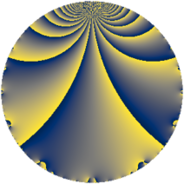# Properties

 Label 342.3.qLevel $342$ Weight $3$ Character orbit 342.q Rep. character $\chi_{342}(83,\cdot)$ Character field $\Q(\zeta_{6})$ Dimension $80$ Newform subspaces $1$ Sturm bound $180$ Trace bound $0$

# Related objects

## Defining parameters

 Level: $$N$$ $$=$$ $$342 = 2 \cdot 3^{2} \cdot 19$$ Weight: $$k$$ $$=$$ $$3$$ Character orbit: $$[\chi]$$ $$=$$ 342.q (of order $$6$$ and degree $$2$$) Character conductor: $$\operatorname{cond}(\chi)$$ $$=$$ $$171$$ Character field: $$\Q(\zeta_{6})$$ Newform subspaces: $$1$$ Sturm bound: $$180$$ Trace bound: $$0$$

## Dimensions

The following table gives the dimensions of various subspaces of $$M_{3}(342, [\chi])$$.

Total New Old
Modular forms 248 80 168
Cusp forms 232 80 152
Eisenstein series 16 0 16

## Trace form

 $$80q + 4q^{3} - 160q^{4} - 8q^{6} - 2q^{7} + 4q^{9} + O(q^{10})$$ $$80q + 4q^{3} - 160q^{4} - 8q^{6} - 2q^{7} + 4q^{9} + 18q^{11} - 8q^{12} - 20q^{13} - 2q^{15} + 320q^{16} + 90q^{17} - 16q^{18} - 22q^{19} + 60q^{21} + 12q^{22} + 16q^{24} + 200q^{25} + 70q^{27} + 4q^{28} + 54q^{29} - 44q^{30} - 8q^{31} - 22q^{33} + 48q^{34} - 8q^{36} - 44q^{37} + 36q^{38} + 78q^{39} + 162q^{41} + 80q^{42} + 88q^{43} - 36q^{44} - 88q^{45} - 72q^{47} + 16q^{48} - 306q^{49} + 432q^{50} + 154q^{51} + 40q^{52} - 72q^{53} + 208q^{54} + 36q^{59} + 4q^{60} - 14q^{61} - 108q^{62} + 64q^{63} - 640q^{64} - 144q^{65} - 96q^{66} - 56q^{67} - 180q^{68} - 136q^{69} - 270q^{71} + 32q^{72} - 104q^{73} - 72q^{75} + 44q^{76} + 432q^{77} + 112q^{78} - 68q^{79} - 260q^{81} + 48q^{82} + 342q^{83} - 120q^{84} + 860q^{87} - 24q^{88} - 216q^{89} + 272q^{90} - 340q^{91} - 224q^{93} - 756q^{95} - 32q^{96} - 212q^{97} - 216q^{98} + 100q^{99} + O(q^{100})$$

## Decomposition of $$S_{3}^{\mathrm{new}}(342, [\chi])$$ into newform subspaces

Label Dim. $$A$$ Field CM Traces $q$-expansion
$$a_2$$ $$a_3$$ $$a_5$$ $$a_7$$
342.3.q.a $$80$$ $$9.319$$ None $$0$$ $$4$$ $$0$$ $$-2$$

## Decomposition of $$S_{3}^{\mathrm{old}}(342, [\chi])$$ into lower level spaces

$$S_{3}^{\mathrm{old}}(342, [\chi]) \cong$$ $$S_{3}^{\mathrm{new}}(171, [\chi])$$$$^{\oplus 2}$$